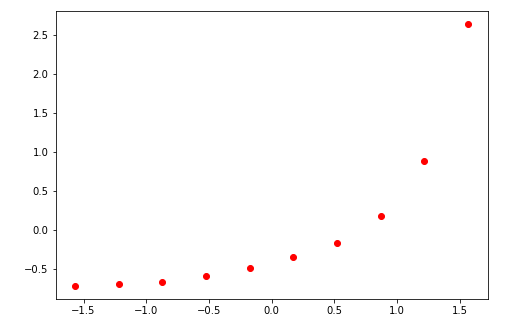top of pageSearch

# A dive into Support Vector Regression with Python

As we are aware that SVM algorithms are mostly used for classification and rarely for regression.

Although it provides us SVR algorithm to do the needful.

In my previous blog I mostly talked about how data can be classified using kernel trick in SVM, here I am going to explain how SVR helps in regression of data.

The objective of Support Vector is to find a hyperplane in N dimensional space which can classify data-points.

The data points lying on the margin and nearest to Hyperplane are called Support Vectors.Now when most of the data lies mostly within the best margin towards each side of hyperplane then SVR or Support Vector Regression can be used to identify and predict the dependent data.It has mathematical formula for marginal lines towards each side of hyperplane is represented by:

Yi = (w , xi) + b + deviation

Yi = (w, xi) + b- deviation

Steps to be followed to build a support regression model:

1) Find your X and Y , independent and dependent data sets to train the model.

2) See the data at glance and try to fit in the best suited kernel parameter. You can also try to plot the data points and see correlation.

It could be linear, Gaussian or Polynomial depending upon the complexity.

The most common kernel used is Gaussian.

•Polynomial kernel: K(x,y) =(x .y +1)d, where d>0 is a constant that defines the kernel

Order.

•Gaussian RBF kernel: K(x,y) =exp(−| x−y|2/2σ2), where σ>0 is a parameter that

Defines the kernel width. The associated parameters d and σ are determined during the training phase.

Now not going too much into theoretical part, let’s see how we can do it in Python.

Step1) Import Libraries

```import pandas as pd
import numpy as np
import matplotlib.pyplot as plt```

Step2) Import dataset

`dataset=pd.read_csv('D:/blog/SVR/PostVsSalary.csv')`

Step 3) Defining X and Y variables i.e. dependent and independent variables.

Here we are taking x as Position level and y as Salary.

```X=dataset.iloc[:,1:2].values
y=dataset.iloc[:,2:].values```

Step 4) Covert the data into Scalar

As we see there is a huge parity between values of Post or Position(1-10) and Salary (45000-1000000), we need to have data on similar grounds for Support Vector Regression model to work.

The standard score of a sample x is calculated as:

Xstandard = (x – mean(x) )/ standard deviation

We do it by using StandardScaler library of Python.

In other linear models we don’t use it as those models have it already in-built but just to do it with our model we need to write following code:

```from sklearn.preprocessing import StandardScaler

st_x=StandardScaler()
st_y=StandardScaler()

X=st_x.fit_transform(x)
Y=st_y.fit_transform(y)```

Step 5) Plot the data to have a look

Plotting Independent data Variables as red dots.

After making the values scalar

```fig=plt.figure()
ax.scatter(X,Y,color='r')```Step 6) Select a kernel to train the data on.

Most important SVR parameter is Kernel type. It can be #linear,polynomial or gaussian SVR.

We have a non-linear condition #so we can select polynomial or gaussian but here we select RBF(a #gaussian type) kernel(parameter='rbf') .

Polynomial kernel takes a lot of complex calculations which takes time for computation.

The Gaussian kernel is the most commonly used kernel in pattern recognition.

```from sklearn.svm import SVR
regressor=SVR(kernel='rbf')
regressor.fit(X,y)```

Step 6) Plot X and Y

```plt.scatter(X, Y, color = 'magenta')
plt.plot(X, regressor.predict(X), color = 'green')
plt.title('PostVsSalary(Support Vector Regression Model)')
plt.xlabel('Post')
plt.ylabel('Salary')
plt.show()```Step 7) Finding out prediction points:

Remember we did scaling of data here. Now if we want to have data back in similar format we need to do is to scale back when we run predictions.

Here, if we want to predict the salary of a level 7.5 position, then we need to create an array with 7.5 and give it to our predict function. Then we will get it inversed to get the scaled back value.

Following code will do the needful.

```# Predicting a new result
X_pred = sc_X.transform(np.array([[7.5]]))
y_scale_back = sc_y.inverse_transform(regressor.predict(X_pred))```

SVR is most suitable for

When we are able to generate training data, we know that what the correct answer. But it may be very expensive if we compute the answer for every new data point need. The SVR, or Gaussian processes, are nice to provide a pocket friendly alternative to an expensive one. If the function we are calling are smooth to compute, then these can be repeatedly called, hence significant savings can be experienced by pre-computing a training set and then using a SVR model to predict the results.

Hope the above blog helps in understanding SVR.